Spitzer Documentation & Tools
IRS Instrument Handbook

### 5.1.2             SNEST_IRS

This module (�Signal-To-Noise Estimate for IRS�) generates, for the first time in the pipeline, the uncertainty of each sample of the data cube. The output file, snest_irs.fits, is a FITS cube of the same dimensions as the data cube. The units are DN. This file is not available from the Spitzer Heritage Archive, but can be created by running CUPID. The computation consists of adding Poisson noise and readnoise in quadrature. With I being the sample value in DN, σreadnoise the readnoise in electrons, and g the gain in electrons/DN , the uncertainty in the sample value is: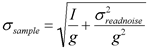Equation 5.1

In deriving the above equation, the variance in electrons due to photon noise is just the sample value I times g. However, the units of this variance are actually electrons2. It should be noted that the parameters, σreadnoise (also denoted �Readnoise_Snest� with default value 40, 33, 67, and 55 electrons for SL, SH, LL, and LH, respectively) and g (also denoted �Gain_Snest� with default value 4.6 electrons/DN), affect the operation of only the current module. They do not affect the input values of readnoise and gain used elsewhere in the pipeline (in the modules DNTOFLUX, RADHIT_SAT, and RADHIT, described below in Sections 5.1.3, 5.1.4 and 5.1.12, respectively).

Before calculating Poisson noise, all samples of a given ramp are offset by adding a constant Δ (unique for each ramp). The purpose of adding Δ is to prevent negative samples or ramps with big offsets from affecting the noise estimation. This offsetting is an internal calculation for SNEST_IRS only and is not propagated. To obtain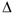, first the absolute values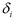of pair-wise sample differences are computed. Denoting the signal at the i-th sample by pi, and the number of samples in the ramp by Nplanes: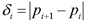Equation 5.2

Here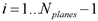.  Then the median of thevalues in the ramp is computed, denoted here by.

Outlier samples pj are identified if their corresponding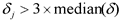.  Excluding these outlier samples, the sample in the ramp with the smallest value is identified, and denoted here by Plowest . See Figure 5.3. The offset to add to all samples of the ramp is: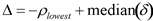Equation 5.3

The minimum non-outlier sample in an offset ramp will thus be, as illustrated in the lower panel of Figure 5.3.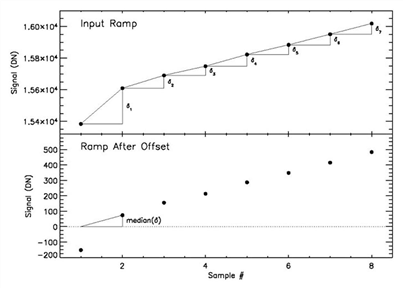Figure 5.3 Before computing Poisson noise, the module SNEST_IRS applies an offset to each input ramp top panel. First, pair-wise differencesfor each sample are computed. Then the median of these (shown in the bottom panel) is used to exclude outlier samples, namely those whose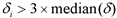. In this case, the first sample is an outlier. Finally, the ramp is offset (bottom panel such that the minimum non-outlier sample (in this case the second sample) has the value. This calculation is internal to SNEST_IRS and is not propagated. Outlier samples get assigned an arbitrarily large Poisson noise value.